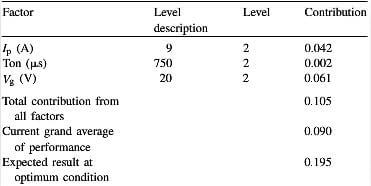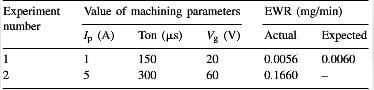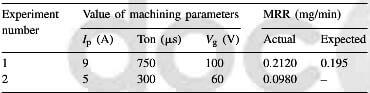# EDM machining of carbon-carbon composite- a Taguchi approach (5)

The optimum conditions of the machining parameters and their levels for optimum MRR are given in Table 9. It shows that for optimum MRR, the levels of the significant factors are their higher levels, i.e., Ip=9A, Vg=100, V Ton=750μs. It is also expected that this combinant of parameters increase the MRR from an average value of 0.09-0.195mm3/min.table-9-optimum-conditions-and-performance-for-MRR

To validate the findings of this analysis, additional experiments are conducted, at the optimum as well as arbitrary levels of the machining parameters, within the region of experimentation and the results are given in tables 10 and 11. The results of the additional experiments show that at the intermediate levels of parameters (i.e., Ip=5A, Ton=300μs and Vg=60V) the EWR is much higher than the expected result at the optimum condition, whereas at the optimum level (Ip=1A, Ton=150μs and Vg=20V) EWR is very close to the expected result, i.e., within 6.67%.table-10-comparison-of-expected-and-actual-values-of-EWRTable 11-comparison of expected and actual values of MRR

Similarly at the optimum level of parameters (i.e., Ip=9A, Ton=750μs and Vg=100V) the MRR is 0.212 mm3/min, which is very close to the expected result of 0.195mm3/min, i.e. within 8.19%, while at the intermediate level (Ip=5A, Ton=300μs and Vg=60V) it is 0.098mm3/min, which is much lower.

This work shows optimisation of the machining parameters in the EDM machining of C-C composite using Taguchi method. The process variables affecting electrode wear rate and MRR, according to their relative significance, are Vg, Ip and Ton, respectively. Among the process parameters Ton is insignificant. The optimum machining conditions forelectrode wear rate, with an expected reduction of 89.28% from the current average value of 0.056mg/min, is Ip=1A, Ton=150μs and Vg=20V. Whereas the same for MRR, with an expected increase of 116.67% from the current average value of 0.09mm3/min, is Ip=9A, Ton=750μs and Vg=100V. The optimum conditions for the two response functions are different. It has been shown that machining parameters set at their optimum levels can ensure significant improvement in the response function, which is confirmed by the additional experiments.